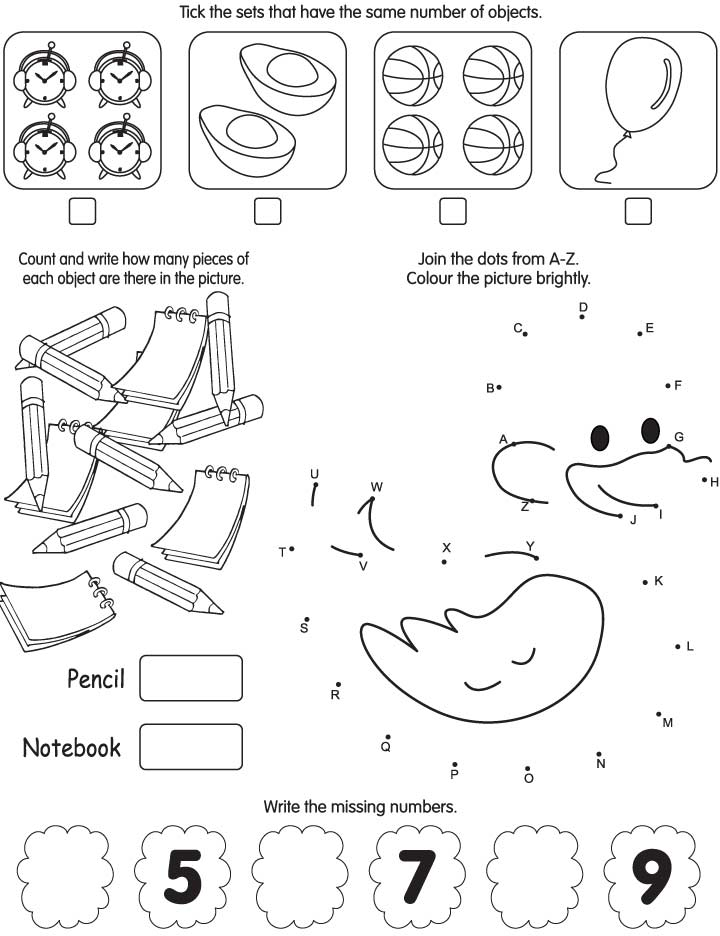# Home

## Worksheet Category

### Simple & Easy Maths Worksheets 95

Page of 20Page of 20

Simple & Easy Maths Worksheet for kids, there are four activities in this page

• Tick the sets that have the same number of objects.
• Count and write how many pieces of each object are there in the picture.
• Join the dots from A to Z. Colour the picture brightly.
• Write the missing numbers.Count and write how manySolve the number puzzlesNumber the picture pieces in the correct orderCount and write starsLook at the three sets of animals belowWrite the numbersComplete the sums using the codesSolve the sumsCross out the following as directedDraw a lineCount and writeFollow the table of 8 and join the starsFind the totalColor the pictures that have even numbersJoin the dots from 1-20Mark the numbers which are not in orderEncircle the number 6 and count how many times it appearsColor the given number of objectsDraw, color and complete the sum